## General Question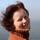# What does this dot in this equation mean?

Asked by Janka (2182) February 8th, 2010

In http://www.cs.mcgill.ca/~icml2009/papers/10.pdf in equation 24a, at the top of page six, in the nominator of the equation inside the log, what does the dot between N and n(ij) mean?

Observing members: 0Composing members: 0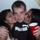I’m fairly certain it’s multiply.

jfos (7365)“Great Answer” (0) Flag as…Response moderated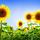Huh, I don’t know either. I hope someone knows, I’m interested to find out the answer too.

JLeslie (58946)“Great Answer” (0) Flag as…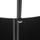@jfos While a dot can mean multiply, in this equation every other term to be multiplied is simply placed next to the term it is to be multiplied by. It doesn’t make sense to use a dot for multiplication once in an entire paper. It’s also down low like a decimal point, whereas a dot used for multiplication is usually higher, at the midpoint of a character. I don’t have any better ideas, but I don’t think that’s it.

Snarp (11259)“Great Answer” (1) Flag as…@Snarp You are nicer than me to even reply to that first respose. I didn’t take it seriously at all.

JLeslie (58946)“Great Answer” (0) Flag as…@Snarp I also noticed that it was low, which is what gave me some doubt. But it may just appear that way because of subscript / superscript…

jfos (7365)“Great Answer” (0) Flag as…I don’t think it’s multiplication either, because of both what @Snarp said and because of the context. A dot product was suggested, but unless I got the whole frigging thing wrong, both N and n(ij) are scalars.

Janka (2182)“Great Answer” (0) Flag as…jfos (7365)“Great Answer” (0) Flag as…@jfos dot and X are used in math at lower levels. Once you are at trig and calc the letters are just side by side, unless something has changed since I was in school, which was 20 years ago. You could be right, maybe whoever wrote the equation could not do a middle dot on their computer, and opted for a dot at the bottom of the line? Hopefully, someone who works with math all of the time will answer. I really thought you were being funny initially.

JLeslie (58946)“Great Answer” (0) Flag as…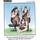could be to the power of or just multiply

talljasperman (21842)“Great Answer” (0) Flag as…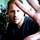Weirder and weirder. Forgetting math notation, I decided to look at the typeface.

It may be intended to be read as a “dot”, but if you copy and paste out the character, you’ll see it’s actually a colon (”:”). The paper shows N.n, but it’s actually N:n.

Normally, I’d guess that the italicized text is unintentionally obscuring the top portion of the character, as can happen sometimes.

However, just a little lower in the page, we see three “dots” together: i=1…R And they’re definitely supposed to be dots in that context, but again, if you copy and paste, you’ll actually get colons: i=1:::R. That’s got to be intentional.

So… I don’t think they’re really supposed to be colons in the mathematical sense. I believe they’re supposed to be “dots” and the author of the doc is just using some typographical sleight of hand for readability.

And if I had to guess, I’d say the dot is there to signify simple multiplication and it’s being done explicitly to ensure there’s no confusion in associating the big N and little n together as a single variable.

robmandu (21280)“Great Answer” (2) Flag as…@robmandu the author probably used a formula editor to create the formulas in the paper, and the PDF may not actually understand what those symbols are, and so for text recognition purposes converts them to colons.

Snarp (11259)“Great Answer” (0) Flag as…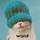Typo, perchance?

shadling21 (6496)“Great Answer” (0) Flag as…@snarp, or… the PDF uses an embedded font that maps that character differently than any I have on my PC… so copy & paste doesn’t really tell the true story.

robmandu (21280)“Great Answer” (0) Flag as…@shadling21 Possible. In which case the question becomes, for what. >_<

Janka (2182)“Great Answer” (0) Flag as…Update: a friend pointed out that a dot is similarly used in (16) where it is explicitly stated to be multiplication. This probably means that my idea that it does not fit the context really is untrue.

I’ll get back to you on this tomorrow.

Janka (2182)“Great Answer” (0) Flag as…And so it appears that for all our conjecture, @jfos was right in the first place. Score one for Occam’s razor.

Snarp (11259)“Great Answer” (1) Flag as…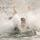@Janka: Glad that you seem to have figured it out!

Note on formatting: The paper was almost definitely typset using LaTeX, a program for typsetting math and science papers (or, as my professor once said, “it’s a gift to the world from the American Mathematical Society”). This means two things: one, if the author wanted a middle dot or a colon, they would have easily been able to put a middle dot or a colon, and the fact that this is a low dot means that, for whatever reason, it’s what the author wanted. Additionally, when LaTeX produces a PDF, it’s not very conducive to copy-pasting into another text editor, so although @robmandu‘s tests were a clever idea, unfortunately they can’t tell us anything here.

All of this is meant to say: I love LaTeX, it’s awesome, and good luck with the project, @Janka!

hannahsugs (3238)“Great Answer” (2) Flag as…@jfos I owe you an apology. Seems you were correct :).

JLeslie (58946)“Great Answer” (1) Flag as…@JLeslie Not to worry.

jfos (7365)“Great Answer” (1) Flag as…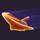It seems to me that the symbol may have been used to distinguish the lower case n from being construed as a subscript of the capital N.

Shuttle128 (2979)“Great Answer” (0) Flag as…It is multiplication.

In the unlikely case that someone’s interested how I convinced myself of this:

In the equation, we are looking at the mutual information of vectors a and b of class assignments (you can think integers) of length N. Let M be the contingency table of those vectors, M = [n(ij)], where i = 1…R and j = 1…C, where R and C are the maximum values of a and b, respectively. We denote by a(i) the number of times value i occurs in a, and with b(j) the number of times j occurs in b.

Now the mutual information is (Latex follows, sorry)
\sum_{i=1}^R \sum_{j=1}^C P(i,j) * log ( P(i,j) / ( P(i)*P(j) ) )

P(i,j) or the probability that values i and j occur together is clearly by the definition of the contingency table n(ij) / N and the marginals P(i) and P(j) are obviously a(i) / N and b(j) / N.

Hence for what is inside the log we have
(n(ij)/N) / ( (a(i)/N) * (b(i)/N) ) =
(n(ij)/N) / ( (a(i)*b(j)) / N^2) =
(n(ij)*N^2) / (N*a(i)*b(j)) =
(N*n(ij)) / (a(i)*b(j)).

Simple brain lock from my side, then. Nothing sleep and coffee don’t solve.

Janka (2182)“Great Answer” (0) Flag as…Oh, and @Shuttle128 is probably right that the dot is included in an attempt to make the Nn(ij) look less confusing. Did not work in my case ;), but I am not a math wiz by any means.

Janka (2182)“Great Answer” (0) Flag as…or